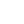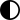DC amps to watts calculation

The power P in watts (W) is equal to the current I in amps (A), times the voltage V in volts (V):

P(W) = I(A) × V(V)

AC single phase amps to watts calculation

The power P in watts (W) is equal to the power factor PF times the phase current I in amps (A), times the RMS voltage V in volts (V):

P(W) = PF × I(A) × V(V)

AC three phase amps to watts calculation

Calculation with line to line voltage

The power P in watts (W) is equal to square root of 3 times the power factor PF times the phase current I in amps (A), times the line to line RMS voltage VL-L in volts (V):

P(W) = 3 × PF × I(A) × VL-L(V)

Calculation with line to neutral voltage

The power P in watts (W) is equal to 3 times the power factor PF times the phase current I in amps (A), times the line to neutral RMS voltage VL-N in volts (V):

P(W) = 3 × PF × I(A) × VL-N(V)

Typhical power factor values

Do not use typhical power factor values for accurate calculations.

 Device Typhical power factor Resistive load 1 Fluorecent lamp 0.95 Incandescent lamp 1 Induction motor full load 0.85 Induction motor no load 0.35 Resistive oven 1 Synchronous motor 0.9

## Comments for Calculating electricity measures

.com

Be the first to comment

Ние се грижим за вашите данни и ще използваме бисквитки само за да подобрим вашето преживяване.
OK, със светла темаOK, с тъмна темаНе желая Декларация за поверителност
!
Сигурни ли сте, че искате да изчистите списъка с любими?
73.74001US